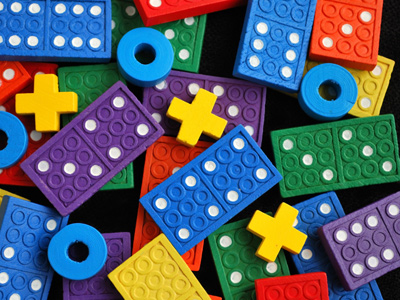# Algebra - Reciprocals 2 - (Moderate - Mixed Numbers)

This Math quiz is called 'Algebra - Reciprocals 2 - (Moderate - Mixed Numbers)' and it has been written by teachers to help you if you are studying the subject at middle school. Playing educational quizzes is a fabulous way to learn if you are in the 6th, 7th or 8th grade - aged 11 to 14.

It costs only \$12.50 per month to play this quiz and over 3,500 others that help you with your school work. You can subscribe on the page at Join Us

A reciprocal is when you have two individual numbers (monomials) that, when multiplied together, will always equal the number “1”. In order for this to occur, the two monomials must be the reverse of each other. For example, let’s look at the monomial fraction 2/6. The reciprocal of 2/6 is 6/2. Now, when we multiply these fractions we get:

2/6 x 6/2 = 12/12
12/12 = 1

That seems easy enough when you have a simple or proper fraction but what if you have a mixed number. See below for examples.

Go straight to Quiz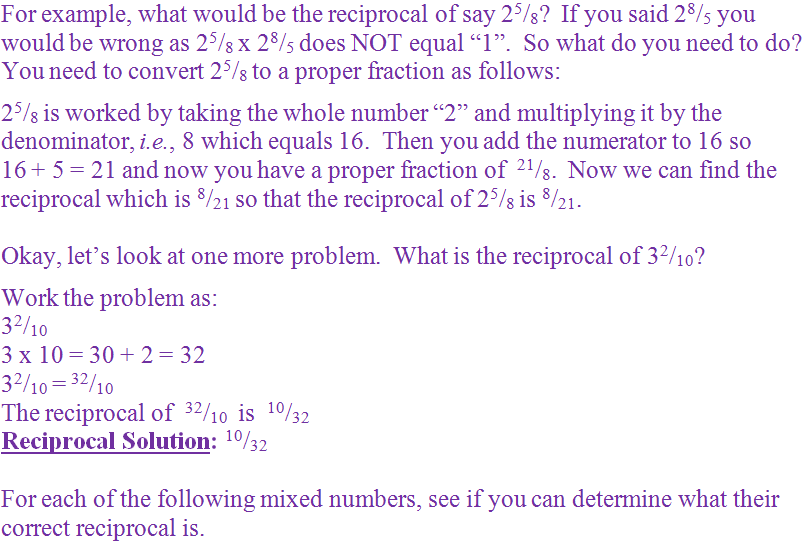1.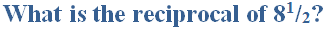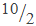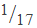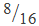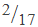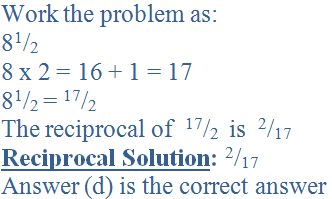2.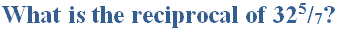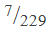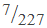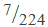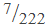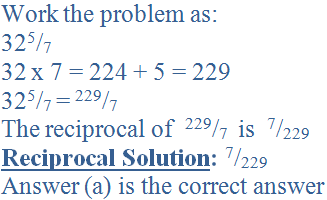3.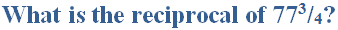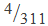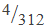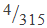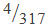4.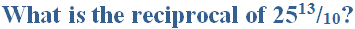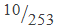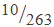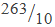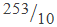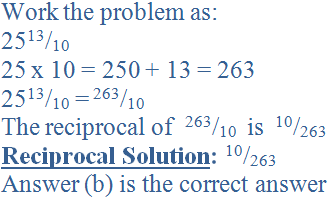5.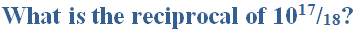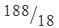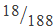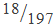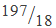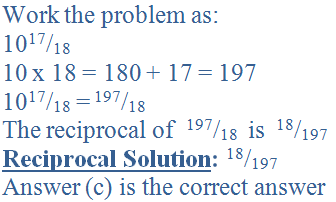6.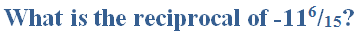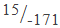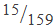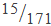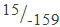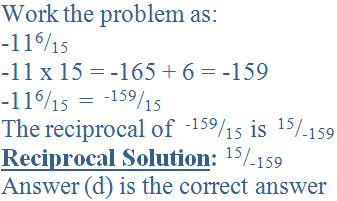7.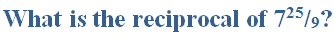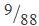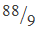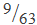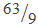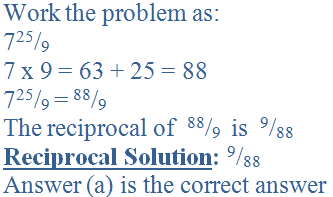8.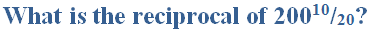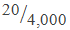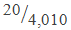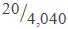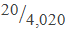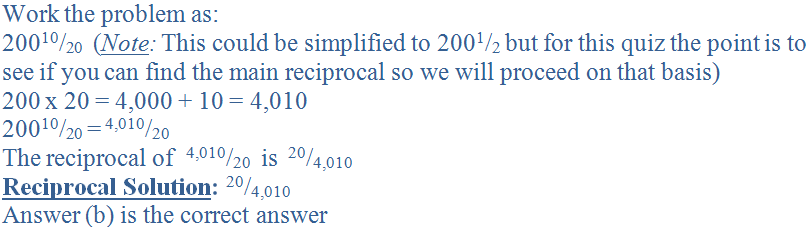9.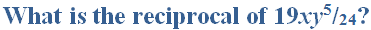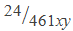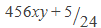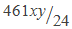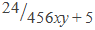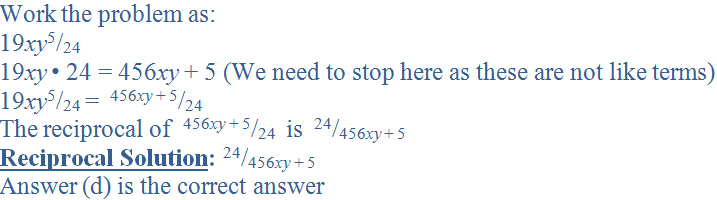10.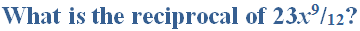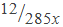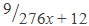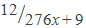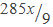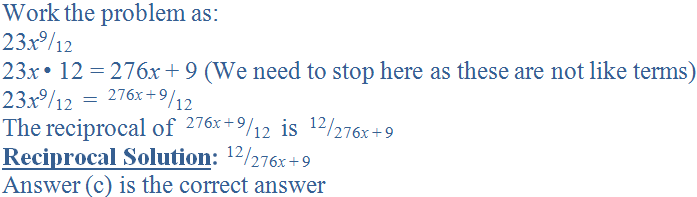Author:  Christine G. Broome